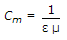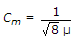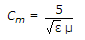# Civil Engineering - Elements of Remote Sensing

### Exercise :: Elements of Remote Sensing - Section 2

31.

Pick up the correct statement from the following :

 A. Frequency is the number of wave crests passing a fixed point in one second B. Frequency of a wave is measured in Hertz (Hz) C. Amplitude of a wave is the height of its crust from the mid-point. D. The square of the amplitude is proportional to the energy transported by the wave. E. All of these

Explanation:

No answer description available for this question. Let us discuss.

32.

If electric permittivity and magnetic permeability of the medium are respectively ε and μ, then the velocity (Cm) of the electromagnetic wave in the medium is given by :

 A.B.C.D. None of these

Explanation:

No answer description available for this question. Let us discuss.

33.

Earth observations from a satellite platform provides :

 A. synoptic view of a large area B. constant solar zenith angles and similar illumination conditions. C. repetitive observations of the same area with intervals of a few minutes to a few weeks D. All of these

Explanation:

No answer description available for this question. Let us discuss.

34.

Due to scan geometery of a satellite sensor:

 A. the off-nadir resolution is degraded B. the ground distance swept by the senor, IFOV is proportional to sec2θ, where θ is the angle of scan measured from the nadir C. the details towards the edge of the scan get compressed D. All of these

Explanation:

No answer description available for this question. Let us discuss.

35.

'A time varrying electric field produces a magnetic field.' This phenemenon is called:

 A. Hertz's law B. Ampere Maxwell's law C. Faraday's law D. Kirchoff s law